# In Figure P6.18 block B weighs 50 lb. Determine the range of values of the weight...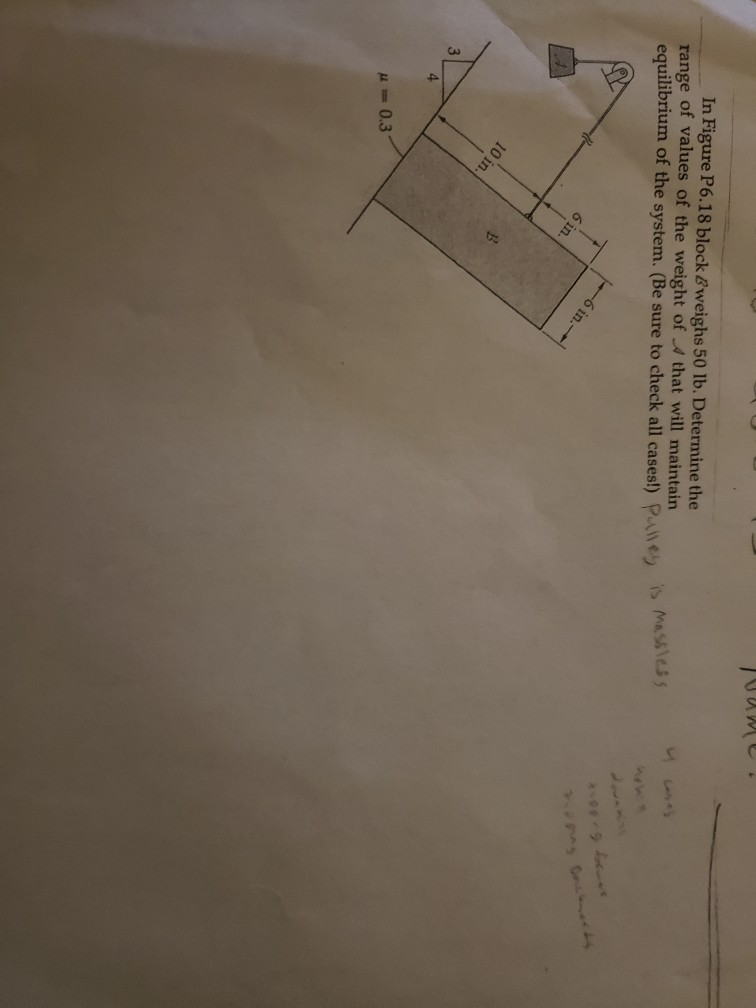In Figure P6.18 block B weighs 50 lb. Determine the range of values of the weight of A that will maintain equilibrium of the system. (Be sure to check all cases!) Pulley leg is massless 10 in. u=0.3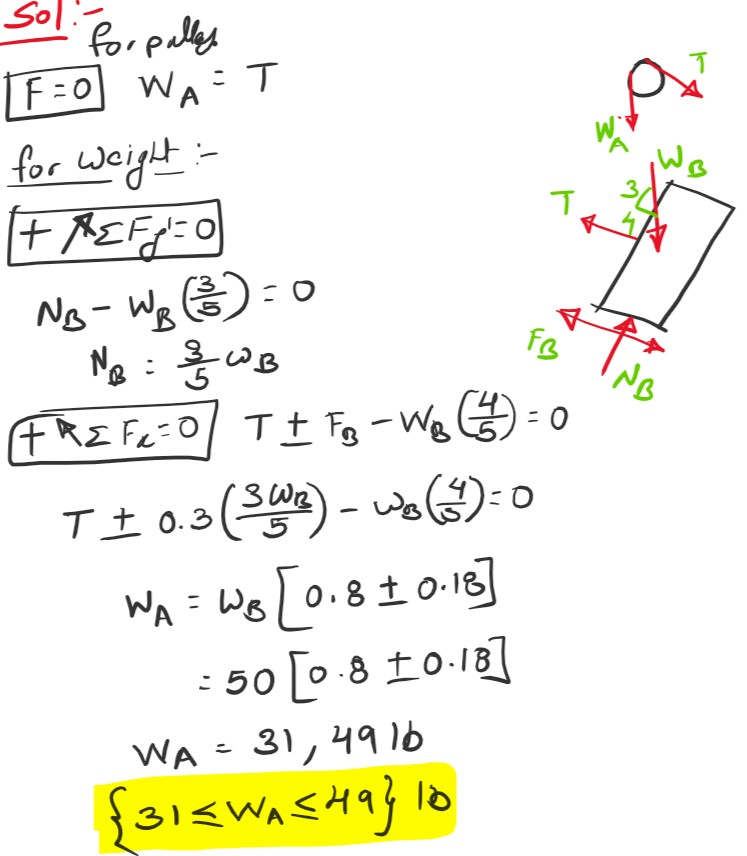#### Earn Coin

Coins can be redeemed for fabulous gifts.

Similar Homework Help Questions
• ### If block B weighs 200 lb and block C weighs 100 lb, determine the required weight...If block B weighs 200 lb and block C weighs 100 lb, determine the required weight of block D and the angle theta for equilibrium. If block D weighs 300 lb and block B weighs 275 lb, determine the required weight of block C and the angle theta for equilibrium.

• ### 1. If block D weights 300 lb and block B weighs 275 lb, determine the required...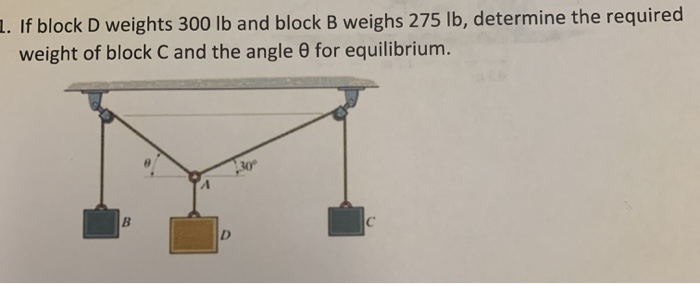1. If block D weights 300 lb and block B weighs 275 lb, determine the required weight of blockC and the angle 0 for equilibrium. 30 B

• ### what is the solution of this problem, If block D weighs 300 lb. and block B...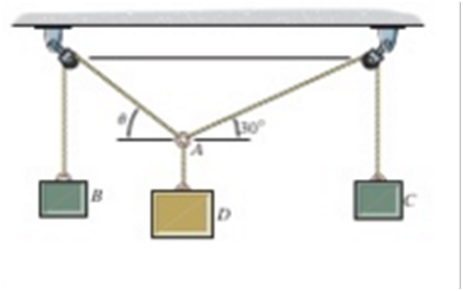what is the solution of this problem, If block D weighs 300 lb. and block B weighs 275 lb. determine the required weight of block C and the angle for equilibrium.

• ### Block A weighs 10 lb and block B weighs 3 lb. If B is moving downward...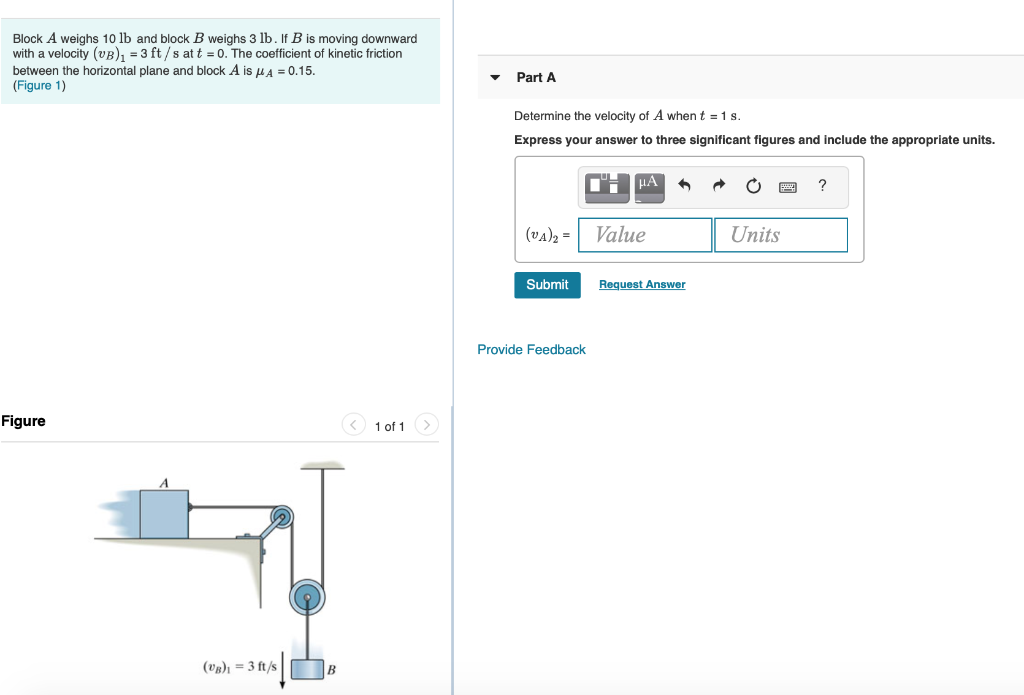Block A weighs 10 lb and block B weighs 3 lb. If B is moving downward with a velocity (UB), = 3 ft/s att = 0. The coefficient of kinetic friction between the horizontal plane and block A is A = 0.15. (Figure 1) Part A Determine the velocity of A when t = 1 s. Express your answer to three significant figures and include the appropriate units. (vs) = Value Units Submit Request Answer Provide Feedback Figure 1 of...

• ### If the sack at 8 in the figure has a weight of 50 lb determine the...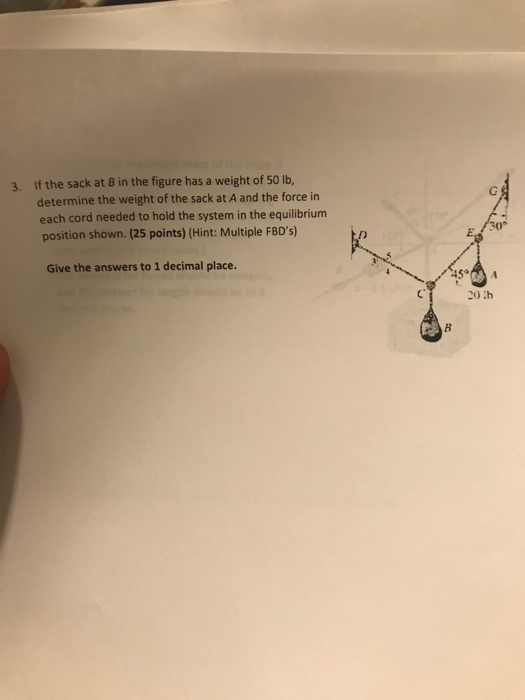If the sack at 8 in the figure has a weight of 50 lb determine the weight of the sack at A and the force in each cord needed to hold the system in the equilibrium position shown. (25 points) (Hint: Multiple FBD's) 3. 30° Give the answers to 1 decimal place 20 th

• ### Consider the system shown in the figure. Block A has weight 4.91 N and block B has weight 2.94 N. Once block B is set in...

Consider the system shown in the figure. Block A has weight 4.91 N and block B has weight 2.94 N. Once block B is set into downward motion, it descends at a constant speed. Assume that the mass and friction of the pulley are negligible. Calculate the coefficient of kinetic friction mu between block A and the table top. I'm not sure where to begin.

• ### Consider the system shown in the figure below. Block A weighs 43.2 N and block B...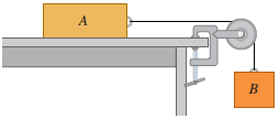Consider the system shown in the figure below. Block A weighs 43.2 N and block B weighs 29.0 N. Once block B is set into downward motion, it descends at a constant speed. (a) Calculate the coefficient of kinetic friction between block A and the tabletop. (b) A cat, also of weight 43.2 N, falls asleep on top of block A. If block B is now set into downward motion, what is its acceleration? magnitude m/s2 direction ---Select---upwarddownward Help!! Consider...

• ### Block A weighs 1.4 N and block B weighs 4.2 N. The coefficient of kinetic friction...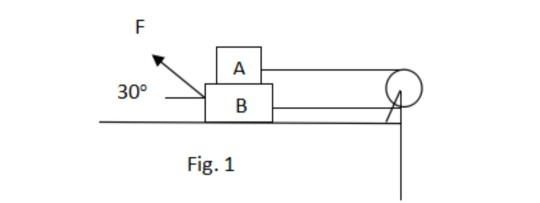Block A weighs 1.4 N and block B weighs 4.2 N. The coefficient of kinetic friction between all surfaces is 0.3. Find the magnitude of force F as shown in Fig. 1 necessary to drag block B to the left at an acceleration of 2 m/s2 if A and B are connected by a light flexible cord passing around a fixed frictionless pulley. F А 30° B Fig. 1

• ### Problem 4 The block D has a weight of 300 lb. The block B has a...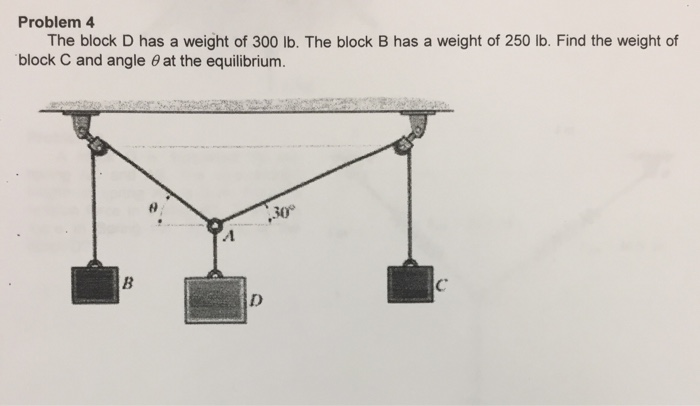Problem 4 The block D has a weight of 300 lb. The block B has a weight of 250 lb. Find the weight of block C and angle O at the equilibrium. 30

• ### Block B in the figure weighs 711 N. The coefficient of staticfriction between the block and...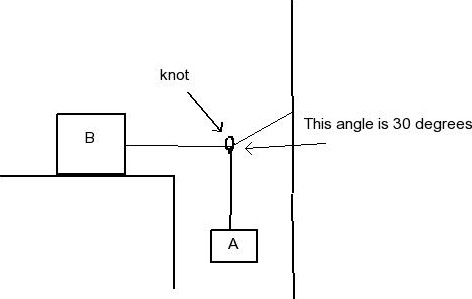Block B in the figure weighs 711 N. The coefficient of staticfriction between the block and table is 0.25. assume that the cordbetween B and the knot is horizontal. Find the maximum weight ofblock A for which the system will be stationary.

Free Homework Help App